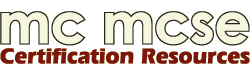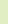The best free certification study guides, practice tests and forums!Join Us! | Login | HelpCertifications Microsoft CompTIA Cisco CIW LPI Red Hat IBM
 Links TechTutorials CertifyPro Certnotes A+ Tutorials# Subnetting 123

Ever get stressed out because you know that there would be subnetting question(s) in the next exam you are taking and that these questions easily take up 10 to 20 minutes of your precious exam time? What if there is more than one question?

The process of converting the subnet to binary and decimal can drive the unfamiliar insane, not to mention the waste of precious time and brain power which can be utilized for other areas of exam preparation.

Let's take a look at a shortcut method that will cut down the time needed to answer these questions without the need for a calculator.

Subnet Basics:

This article assumes that you know how to perform subnetting in the traditional method but it is important to stress that there are only 3 classes of usable IP addresses which are:

 Class Range Subnet mask Host bit Subnet Class A 1 - 126 (127 is reserved for loopback) 255.0.0.0 24 8 Class B 128 - 191 255.255.0.0 16 16 Class C 192 - 223 255.255.255.0 8 24

You must understand and remember this table well in order to master the shortcut.

Note: You must borrow at least 2 bits and must leave at least 2 bits.

The Subnet Table:

 Bits Borrowed (N) 1 2 3 4 5 6 7 8 Bit Value 128 64 32 16 8 4 2 1 Subnet Mask 128 192 224 240 248 252 254 255 Number of Subnets ((2^N)-2) 0 2 6 14 30 62 126 254

Stop: Before continuing, please read this very important article regarding the use of the ((2^N)-2) method of subnetting.

If using the (2^N) method as defined in RFC 1878, your table would look like this instead.

 Bits Borrowed (N) 1 2 3 4 5 6 7 8 Bit Value 128 64 32 16 8 4 2 1 Subnet Mask 128 192 224 240 248 252 254 255 Number of Subnets(2^N) 2 4 8 16 32 64 128 256

The subnet table is commonly seen in lecture notes or certification guides but what the author/lecturer did not tell you is how to derive this table on the fly. Its actually quite simple, lets look at it line by line.

1. Bits borrowed, this is the easy one, just remember that the table consists of only 8 columns.
2. Bits Value, remember by heart that the first value starts with 128 and the subsequent values are divided by two.
3. Subnet Mask, this line tells you what the subnet mask would be, to get the figures, add up the corresponding bits value and all of the values prior to it.

4. 128 + 0 (there is no prior value) =128
128 + 64 = 192
192 + 32 = 224
224 + 16 = 240
240 + 8 = 248
248 + 4 = 252
252 + 2 + 254
254 + 1 + 255

5. Number of Subnets, tells you how many subnet you'll get if you use the subnet mask. Just look at the corresponding N value at the top and you can derive the figures.
Once you understand how to derive the subnet table, spend some time practicing. I would advise you to draw out the table once you are in the exam room (before starting the actual exam) it will take you less than a minute.

How to Tackle the Questions:

There are only a few different ways that Microsoft or Cisco can phrase their questions, lets take a look at some examples:

Question Type 1:
If you are to determine the subnet mask based on a number of hosts and an IP address...

Example:
1. Determine the number of bits needed for the hosts. In this scenario, we need ten bits as 2^10 = 1024 (the question asks for 1000 hosts only).
2. Determine the number of bits left for the subnet. 32 - (number of bits needed for the host) which is 32-10 = 22 bits.
3. Determine the number of bits actually borrowed. We take the number of bits left for the subnet and minus as many 8s as possible as each 8 represents 1 octal. Therefore 22 - 8 - 8 = 6 bits were borrowed.
 Bits Borrowed (N) 1 2 3 4 5 6 7 8 Bits Value 128 64 32 16 8 4 2 1 Subnet Mask 128 192 224 240 248 252 254 255 Number of Subnets(2N-2) 0 2 6 14 30 62 126 254

With reference to the subnet table, 6 bits would have a subnet of 255.255.252.0 . Take note that a total of two 8s were subtracted off, therefore the first two octal would be 255.255.x.x and the 3rd octal was 6 bits borrowed which leaves with 255.255.252.x.

Question Type 2:
If you were given an IP address of 172.30.0.0 and you need 15 subnets...

 Bits Borrowed (N) 1 2 3 4 5 6 7 8 Bits Value 128 64 32 16 8 4 2 1 Subnet Mask 128 192 224 240 248 252 254 255 Number of Subnets(2N-2) 0 2 6 14 30 62 126 254

With reference to the subnet table, the subnet mask should be 255.255.248.0. 172.30.0.0 is a Class B address and the subnet should be 255.255.0.0.

Question Type 3:
You are assigned an IP address of 172.30.0.0 and you need 55 subnets, how many hosts do you have per subnet?
1. Determine the number of bits used for the subnet.

 Bits Borrowed (N) 1 2 3 4 5 6 7 8 Bits Value 128 64 32 16 8 4 2 1 Subnet Mask 128 192 224 240 248 252 254 255 Number of Subnets(2N-2) 0 2 6 14 30 62 126 254

According to the chart, the closest match to 55 subnet would be 62 and therefore, the number of bits borrowed for the subnet is 6. Since 172.30.0.0 is a Class B, we would need to add another 16 bits to the 6 making it 22 bits in total.
2. Determine the number of bits used for the host. Number of bits used for the hosts is 32 - (number of bits used for the subnet) which is 22 = 10 bits. 2^10-2 = 1022, therefore there are a total of 1022 usable hosts in each subnet.
The key to mastering this shortcut is the same as with any other mathematical question - pratice.

Good luck on your next exam.

By Adam Chee W.S. (MCP ID 2915249)Sponsors